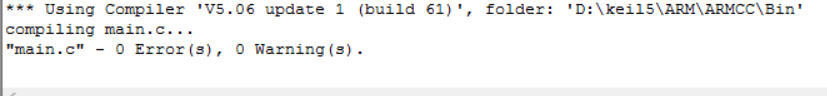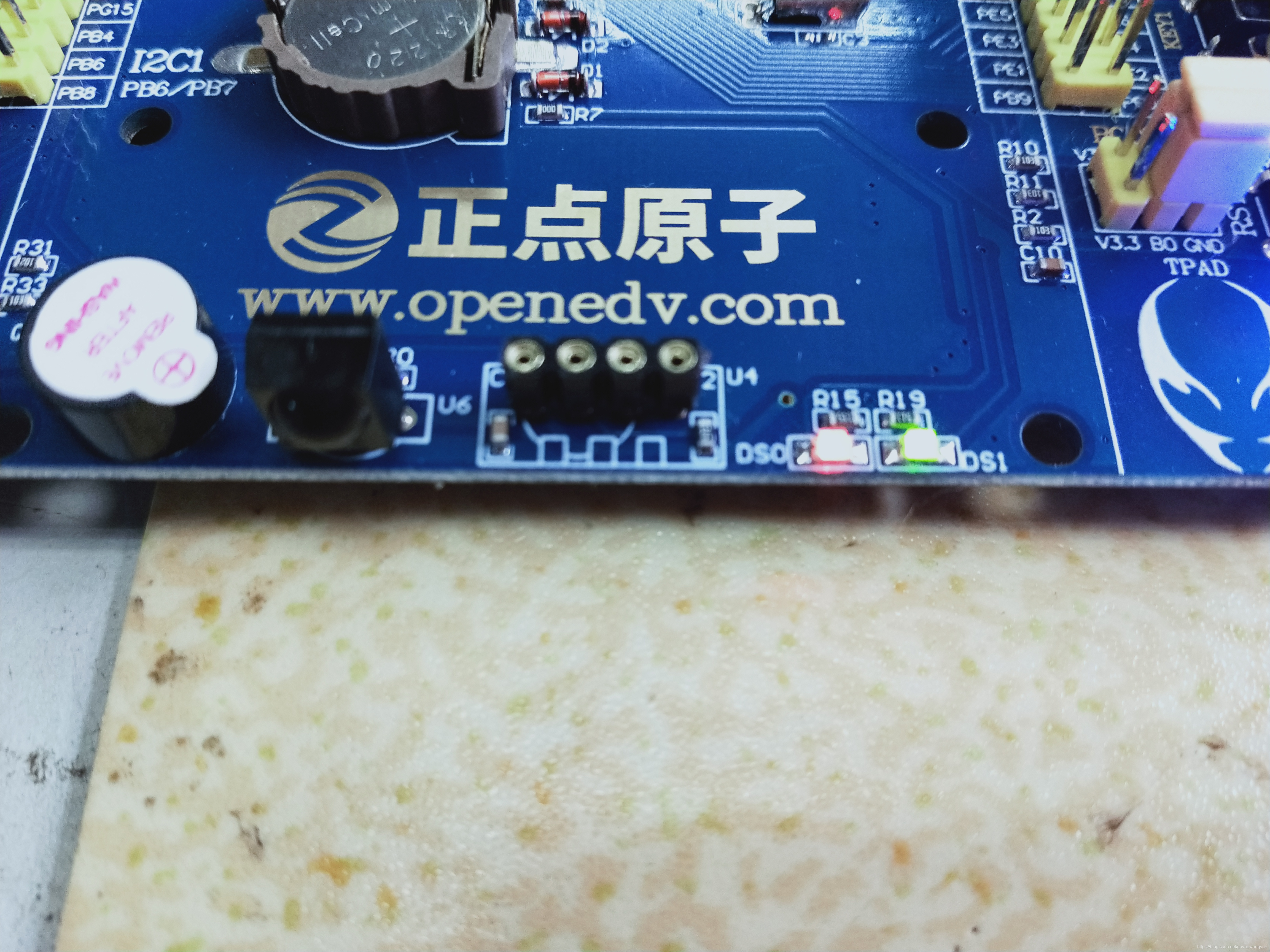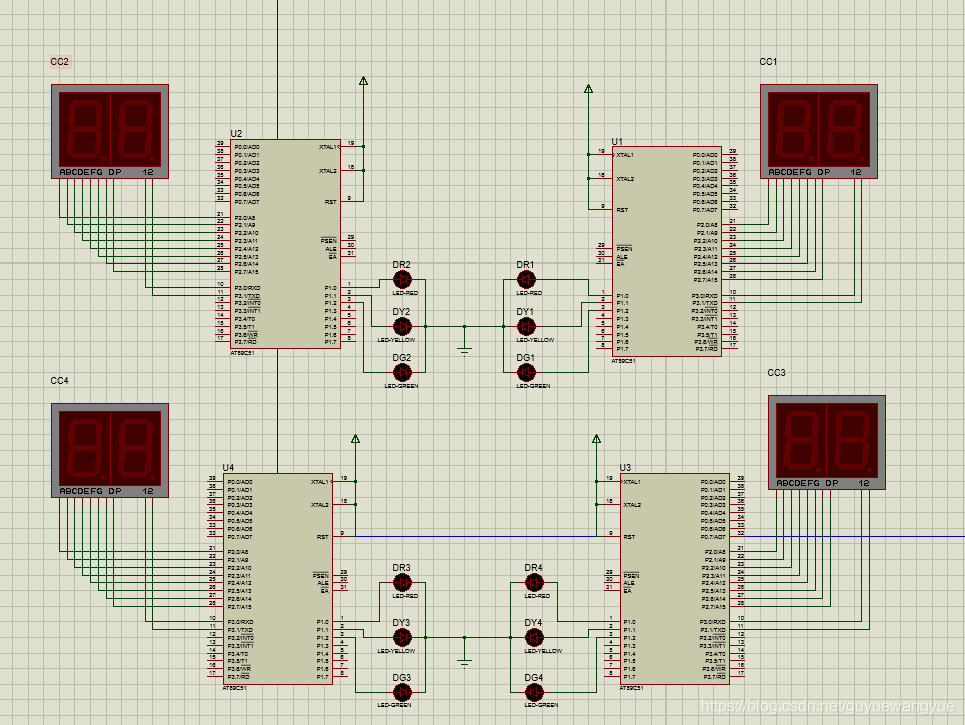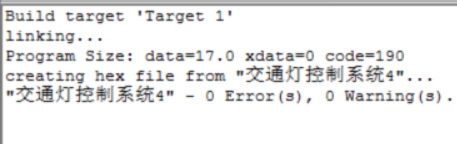• 首页
• 论坛
• 版块

• 问答
• 活动
• 下载
• 学院
• 发现

• 工作
• 升级VIP

[问答]

MDK5及Proteus开发环境如何入门？64
2021-11-11 07:55:19评论淘帖 邀请回答

相关推荐

< >
1个回答
 前言   想要学习ARM的嵌入式开发，就得先熟悉ARM的开发环境。本文讲到的开发环境就包括MDK5和proteus电路仿真软件。本文将简单的熟悉软件的操作，完成一个简单的仿真。 MDK5   创建keil MDK5工程文件可以参考：https://blog.csdn.net/qq_42041980/article/details/85079185 主函数： #include "delay.h" #include "led.h" int main(void) {                delay_init();            //延时函数初始化                   LED_Init();                          //初始化与LED连接的硬件接口         while(1)         {                 LED0=0;                 LED1=0;                 delay_ms(300);         //延时300ms                 LED0=1;                 LED1=1;                 delay_ms(300);        //延时300ms         } } 编译结果：下载实物显示：红色黄色两个LED灯同时闪烁，即同亮同灭。 Proteus   本文使用的proteus版本为proteus8 proteus工程的创建可以参考：https://www.cnblogs.com/caishunzhe/p/12313669.html proteus中的连线如图所示：keil中的代码如下： #include #define uchar unsigned char sbit p10=P1^0; sbit p11=P1^1; sbit p12=P1^2; uchar j,k,i,m,a,b,s,q; unsigned char code tab={0x3f,0x06,0x5b,0x4f,0x66,0x6d,0x7d,0x07,0x7f,0x6f}; void delay(uchar i) {         for(j=i;j>0;j--)         for(k=198;k>0;k--); } void display(uchar sh_c,uchar g_c) {         P3=0x01;         P2=tab[sh_c];         delay(5);         P3=0x02;         P2=tab[g_c];         delay(5); } void main() {         s=28;         p10=0;p11=0;p12=1;         q=s;         while(1)         {                 if(q==31&&s==1)                 {                         s=28;                         p10=0;p11=0;p12=1;                         q=s;                 }                 if(q==28&&s==1)                 {                         s=4;                         p10=0;p11=1;p12=0;                         q=s;                 }                 if(q==4&&s==1)                 {                         s=31;                         p10=1;p11=0;p12=0;                         q=s;                 }                 s--;                 a=s%10;                 b=s/10;                 for(m=200;m>0;m--)                 {                         display(a,b);                 }         } } 编译结果：需要将keil中生成的hex文件放入单片机中，才能有运行结果 仿真结果：   时钟开始倒计时，根据倒计时显示红绿灯。 总结   1、想要编写stm32的程序，就需要在keil MDK5中安装相应的包，同样，想要编写51单片机的程序也需要下载51单片机的包。 2、想要用实物来显示，是需要连接实物之后，将程序下载到板子上面；如果是用来仿真，就需要在仿真的板子上面加入程序文件，才能显示出程序作用后的结果。 keil MDK5和protues 中都是用C语言来编写程序的。 开发环境都需要我们慢慢来熟悉，谁也不能一蹴而就，一起加油吧！

创建小组步骤

•最新主题
•推荐主题
•热门主题
•我的帖子
-

-

-

-

-

-

-

-

-

-

-

-